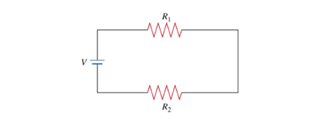# Problem: Two resistors in SeriesConsider the setup shown of two ideal ohmic resistors. (Figure 1).Resistor 1 has resistance R1, and resistor 2 has resistance R2. They are connected in series with a constant voltage of magnitude V. When the two resistors are connected in this way, they form a system equivalent to a single resistor of resistance R, as shown in the next diagram. (Figure 2).Part AWhat is the effective resistance R of the two-resistor system?Express the effective resistance in terms of R1 and R2.

###### FREE Expert Solution

Equivalent resistance for resistors in series:

$\overline{){{\mathbf{R}}}_{{\mathbf{eq}}}{\mathbf{=}}{{\mathbf{R}}}_{{\mathbf{1}}}{\mathbf{+}}{{\mathbf{R}}}_{{\mathbf{2}}}{\mathbf{+}}{\mathbf{.}}{\mathbf{.}}{\mathbf{.}}{\mathbf{+}}{{\mathbf{R}}}_{{\mathbf{n}}}}$###### Problem Details

Two resistors in Series

Consider the setup shown of two ideal ohmic resistors. (Figure 1).

Resistor 1 has resistance R1, and resistor 2 has resistance R2. They are connected in series with a constant voltage of magnitude V. When the two resistors are connected in this way, they form a system equivalent to a single resistor of resistance R, as shown in the next diagram. (Figure 2).Part A

What is the effective resistance R of the two-resistor system?

Express the effective resistance in terms of R1 and R2.